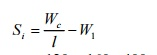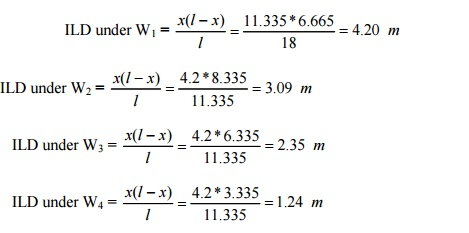Home | | Structural Analysis II | Solved Problems: Structural Analysis- Influence lines

# Solved Problems: Structural Analysis- Influence lines

Civil - Structural Analysis - Influence lines

1.A simply supported beam of span 10m carries a udl of 20 kN/m over its central 4m length. With the help of influence line diagram, find the shear force at 3m from the left support.2.A  single  rolling  load  of  100  kN  moves  on  a  girder  of  span  20m.

(a) Construct the influence lines for (i) shear force and (ii) bending moment for a section 5m from the left support. (b) Construct the influence lines for points at which the maximum shears and maximum bending moment develop. Determine these values.

Solution:

To find maximum shear force and bending moment at 5m from the left support:

(i)  Maximum positive shear force

By inspection of the ILD for shear force, it is evident that maximum positive shear force occurs when the load is placed just to the right of D.

Maximum positive shear force = load * ordinate = 100 * 7.5

At D, SFmax + = 75 kN. (ii) Maximum negative shear force

Maximum negative shear force occurs when the load is placed just to

the left D.

Maximum negative shear force = load * ordinate = 100 * 0.25 At D, SFmax = -25 kN.

(iii) Maximum bending moment

Maximum bending moment occurs when the load is placed on the section D itself.

Maximum bending moment = load * ordinate = 100 * 3.75 = 375 kNm

(b)             Maximum positive shear force will occur at A. Maximum negative shear force will occur at B. Maximum bending moment will occur at mid span. The ILs are sketched in fig.

(i)                Positive shear force

Maximum positive shear force occurs when the load is placed at A. Maximum positive shear force = load * ordinate = 100*1

SFmaxmax + = 100 kN (ii) Negative shear force

Maximum negative shear force occurs when the load is placed at B. Maximum negative shear force = load * ordinate = 100 * (-1)

SFmaxmax = - 100 kN (iii) Maximum bending moment

Maximum bending moment occurs when the load is at mid span Maximum bending moment = load * ordinate = 100 * 5 = 500 kNm

3.Draw the ILD for shear force and bending moment for a section at 5m from the left hand support of a simply supported beam, 20m long. Hence, calculate the maximum bending moment and shear force at the section, due to a uniformly distributed rolling load of length 8m and intensity 10 kN/m run.(Apr/May 05)

Solution:

(a) Maximum bending moment:

Maximum bending moment at a D due to a udl shorter than the span occurs when the section divides the load in the same ratio as it divides the span.(b) Maximum positive shear force

Maximum positive shear force occurs when the tail of the UDL is at D as it traverses from left to right.(c) Maximum negative shear force

Maximum negative shear force occurs when the head of the UDL is at D as it traverses from left to right.

Maximum negative shear force = Intensity of load * Area of ILD under the load = 10(1/2*0.25*5)

Negative SFmax = 6.25 kN.

4.Two point loads of 100 kN and 200 kN spaced 3m apart cross a girder of span 15m  from  left  to  right  with   the  100  kN  load  loading.  Draw the influence line for shear force and bending moment and find the value of maximum shear force and bending moment at a section, 6m from the left hand support. Also, find the absolute maximum moment due to the given load system.5.A train of 5 wheel loads crosses a simply supported beam of span 22.5 m. Using influence lines, calculate the maximum positive and negative shear forces at mid span and absolute maximum bending moment anywhere in the span.

Solution:

(a)             Maximum shear force

(i)                Positive shear force

To determine the load position to get the maximum positive shear force, let us keep all the loads to the right of C. Then move W1 load to the left of 'C'by2.5 m. if the sign of shear increments Si is negative, it will indicate that W1 shall be retained at CW = Total load on the span = 120 + 160 +400 + 260 +240 = 1182 kN.

C = Distance through which the load train is moved = 2.5 mSince Si is positive, the shear force increases due to thr shifting of W1 to the left of C. Again, let us move W2 to the left of C by 2.5 m to check whether the shera force further increases or not.Since Si is negative, it undicates that to get maximum positive shear force, W2 should stay just right of C.Maximum positive shear force = (laodxordinate)

= 120 (-0.39) + 160 (0.5) +400 (0.39) +260(0.28) + 240 (0.17)

At C ,                SFmax + = 302.8 kN.

ii) Negative shear force

To determine the position of loads to get the maximum negative shear force, move the loads one by one to the right of C and computer the value of Si.If Si becomes negative it will indicate a decrease in negative shear force due to that movement.

First let us move the leading W5 to the right of C by 2.5 m and calculate SiSince Si is -ve it indicates that W5 should stay just to the left of C.

Ordinates of ILD:Maximum negative shear force at C = 240(-0.5)+260(-0.39)+(400(-0.278) +160(-0.167)

+120(-0.056)

Fmax = 366.04 kN.

b)                Absolute maximum bending moment

i)                   Position of resultant of all loads

-

Taking moments about W1, 120(0)+160(2.5)+400(5.0)+260(7.5)+240(10.0) =R. x

R = 1180kN

-

x                                                                                                                                    = 5,72m from W1

ii)                                      Location of absolute maximum bending moment

Absolute maximum bending moment occurs under the load, which is nearest to the resultant (In'R'thisproblem. W3 is nearest to the resultant 'R').The distance between C and R and the distance between C and W3 shall be equal.

Distance between R and center of span(C) = � (0.72) =0.36 m

In this fig. Shows the IL for bending moment at the critical spot D, 10.89 m fro A.

Ordinates of ILD:

Maximum ordinate of ILDAbsolute maximum bending moment =120 (3.04)+160(4.33)+400(5.62)+260(4.41)+ 240 (3.2)

Mmax max = 5220.2 kN.m

6) A girder a span of 18mis simply supported at the ends. It is traversed by a train of loads as shown in fig. The 50 kN load loading. Find the maximum bending moment which can occur (i) under the 200 kN load (ii) Under 50 kN load, using influence line diagrams.

Solution:

a)               Maximum bending moment

i)                       Under   200   kN

To get the maximum bending moment under W3 the resultant R and W3 should be at equal distances from the center of the span C.For that the point of action of resultant R should be determined first.

R = 450 kN

W4

200(3) +100 (3+2) +50(3 +2+3) = 450  x

-

x  = 3.33 from W4

b)  Bending moment under 200 kN load

Distance between C and 200 kN load = Distance between C and R = 0.33/2 = 0.165 m.

Ordiantes of ILD :=2.05 m

Bending moment under the 200 kN load

=                                                               200 (4.5) + 100 (2.97) + 100 (3.52) +50 (2.05) = 1651.5 kNm.

To get the maximum bending moment under W1 , W2 and R must be at equal distances from the centre of span �. Distance between C and R = Distance between C and W1 = � (2 -0.33 + 3) = � (4.67) = 2.335 m

Ordinates of ILD:= 4.2 (50) + 3.09 (100) +2.35 (200) +1.24 (100) = 1113 kN m.

6.Draw the I.L for reaction at B and for the support moment MA at A for the propped cantilever in fig. Compute the I.L ordinates at 1.5 m intervals.

Solution:

Remove the restraint due to RB (remove support B) Apply a unit displacement (upward).

When RB = 1, then YXB is the displacement at section x due to unit load applied at B.Fig. is the influence line diagram for RB.

To get the I.L for MA have to

(i)                      Introduce a hinge at A and

(ii)                   We have to apply a unit rotation at A. Instead we will apply a unit moment at A find the general displacement at any x from B. We will then divide the displacement by the actual rotation at A.7.                             In a simply supported girder AB of span 2om, determine the maximum bending moment and maximum shear force at a section 5m from A, due to the passage of a uniformly distributed load of intensity 20 kN/m, longer than the span.

Solution:

(i) Maximum bending moment

Since the udl is longer than the , the criterion for maximum bending moment at a section is that the whole span should be loaded as shown in fig.

(ii) Maximum shear force

Maximum negative shear force at a section occurs when the head of the load reaches the section ((i.e. when the left portion AX is loaded and right portion XB is empty)

(iii)                 Maximum positive shear force:

Maximum positive shear force occurs at X when the tail of the load is at X as it moves from left to right. (i.e. AX is empty and the portion XB is loaded)

Maximum positive shear force = RA

Study Material, Lecturing Notes, Assignment, Reference, Wiki description explanation, brief detail
Civil : Structural Analysis : Influence lines : Solved Problems: Structural Analysis- Influence lines |